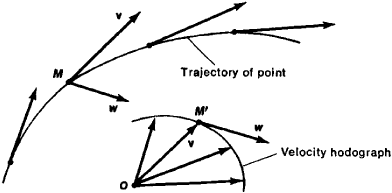# Hodograph

(redirected from hodographic)
Also found in: Dictionary.

## hodograph

[′häd·ə‚graf]
(physics)
The curve traced out in the course of time by the tip of a vector representing some physical quantity.
In particular, the path traced out by the velocity vector of a given particle.
McGraw-Hill Dictionary of Scientific & Technical Terms, 6E, Copyright © 2003 by The McGraw-Hill Companies, Inc.
The following article is from The Great Soviet Encyclopedia (1979). It might be outdated or ideologically biased.

## Hodograph

in mechanics, a curve that is a locus of the ends of a variable vector whose values at different moments of time are laid off from a fixed origin O (see Figure 1).Figure 1. Construction of velocity hodograph

The concept of the hodograph was introduced by the English scientist W. Hamilton. The hodograph visually and geometrically represents the changes with time of a physical quantity, represented by a variable vector, and the rate of this change, which has the same direction as the tangent to the hodograph. For example, the velocity of a point is a quantity represented by the variable vector v. Plotting the values of vector v from the origin O at different moments of time, we derive the velocity hodograph. The quantity characterizing the rate of change in velocity at point M (that is, the acceleration w at point M) for any moment of time has the same direction as the tangent to the velocity hodograph at the corresponding point (M’).

S. M. TARG